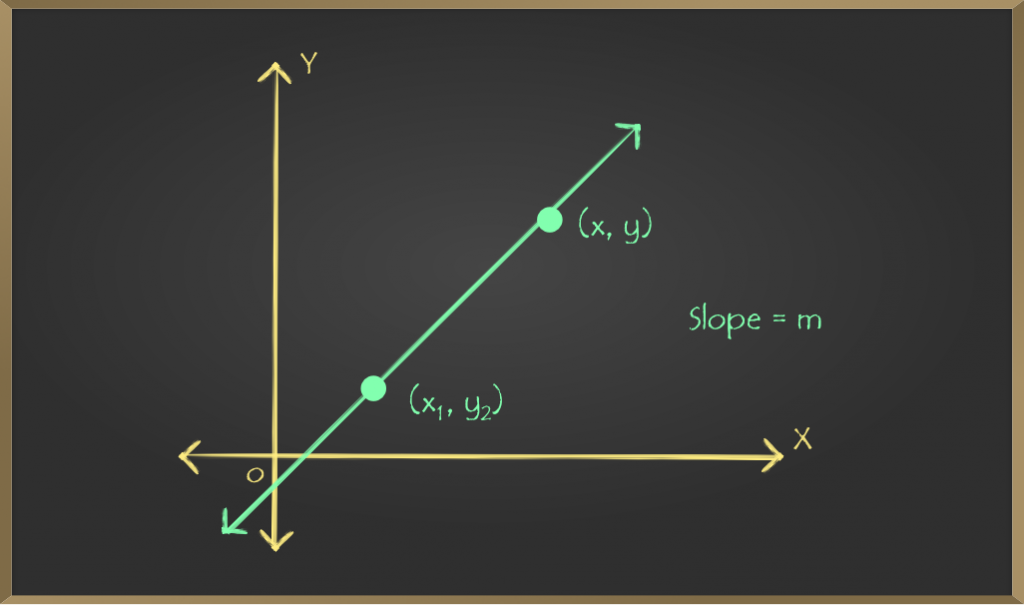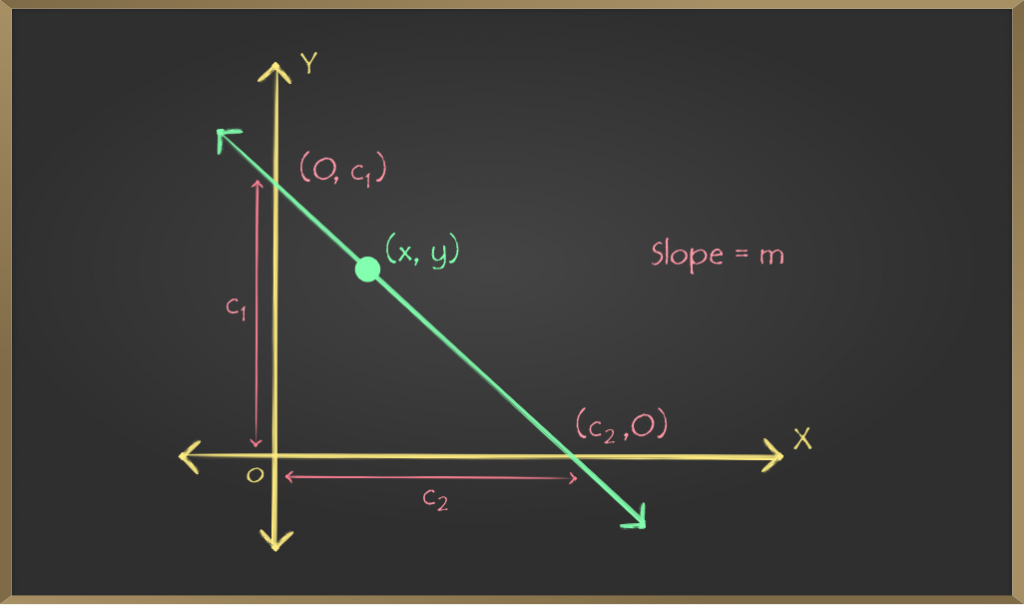GFG App
Open AppBrowser
Continue

# Point-slope Form – Straight Lines | Class 11 Maths

There are several forms to represent the equation of a straight line on the two-dimensional coordinate plane. Three major of them are point-slope form, slope-intercept form, and general or standard form. The point-slope form includes the slope of the straight line and a point on the line as the name suggests. There can be infinite lines with a given slope, but when we specify that the line passes through a given point then we get a unique straight line. Thus, only a point on the line and its slope is required to represent a straight line in the point-slope form.

## Point-slope equation

Consider any straight line on the two-dimensional coordinate plane. Let its slope be m and (x1, y1) be a fixed point lying on the line. There can be infinite lines with slope m but when we specify that the line passes through the fixed point (x1, y1) then we get a unique straight line. Let (x, y) be any arbitrary point on the line. We know the slope of the line is m, i.e. slope between any two points lying on the line will always be m. Using this fact we can derive the equation of any straight line with a given slope and passing through a fixed point.We know,

(change in y) / (change in x) = slope,

Since (x, y) and (x1, y1) are two points on the line and m is the slope of the straight line, slope between (x, y) and (x1, y1) will be equal to m.

Now, slope between (x, y) and (x1, y1) = (y – y1) / (x – x1)

∴ (y − y1) / (x − x1) = m               …(1)

Multiplying both sides of equation (1) by (x − x1) we get,

(y − y1) = m (x − x1)               …(2)

which is the equation for the considered straight line or the point-slope form.

The reason this equation is called the point-slope form is very obvious. The equation only contains the slope and the fixed point on the straight line as constants, so it is called point-slope form.

### Sample Problems on Point-slope Equation

Problem 1: Find the equation of the straight line with slope 3 and
passing through (-1, 5).

Solution:

Here slope of the line is 3 or m = 3 and the line passes through (-1, 5),

hence a fixed point on the line is

(-1, 5) or (x1, y1) = (-1, 5)

Let (x, y) be any point on the line

∴ Slope between (x, y) and (x1, y1) = (y – y1) / (x – x1)

Since (x1, y1) and (x, y) are two points on the line,

the slope between them will be the slope of the line (m)

∴ (y – y1) / (x – x1) = m

Multiplying both sides by (x – x1) we get,

⇒ y – y1 = m(x – x1)

Putting m = 3 and (x1, y1) = (-1, 5) we get,

⇒ y – 5 = 3(x – (-1))

⇒ y – 5 = 3(x + 1)

⇒ y – 5 = 3x + 3

⇒ y – 3x – 5 – 3 = 0

⇒ y – 3x – 8 = 0, which is the required equation

Problem 2: Find the equation of the straight line with slope -2 and passing through (7, -4).

Solution:

Here slope of the line is -2 or m = -2 and the line passes through (7, -4), hence a fixed point on the

line is (7, -4) or (x1, y1) = (7, -4)

Let (x, y) be any point on the line

Since (x1, y1) and (x, y) are two points on the line, the

slope between them will be the slope of the line

∴ (y – y1) / (x – x1) = m

Putting m = 3 and (x1, y1) = (7, -4) we get,

⇒ (y – (-4)) / (x – 7) = -2

Multiplying both sides by (x – 7) we get,

⇒ y + 4 = (-2)(x – 7)

⇒ y + 4 = -2x + 14

⇒ y + 2x + 4 – 14 = 0

⇒ y + 2x – 10 = 0, which is the required equation

Problem 3: Find the equation of the straight line with slope 1/4 and passing through (2, 3).

Solution:

Here slope of the line is 1/4 or m = 1/4 and the line passes through (2, 3),

hence, a fixed point on the line is (2, 3) or (x1, y1) = (2, 3)

We know, point-slope form is:  (y – y1) = m(x – x1)

Putting the values of m, x1 and y1 in the equation we get,

⇒ (y – 3) = (1/4)(x – 2)

Multiplying both sides by 4 we get,

⇒ 4(y – 3) = 1(x – 2)

⇒ 4y – 12 = x – 2

⇒ 4y – x – 12 + 2 = 0

⇒ 4y – x – 10 = 0, which is the required equation

## Slope-Intercept Equation

Slope-intercept form can be treated as a special case of the point-slope form where the fixed point on the straight line lies on X-axis or Y-axis. When a straight line passes through any of the axes, it cuts the axis at a fixed point at a certain distance from the origin. This distance is called the intercept of the line. So if the intercept is given, we can easily determine the cutting point and use it as the fixed point in point-slope form. If the line is said to cut the Y-axis on a distance of c1 from the origin, then it is clear that the cutting point is on Y-axis and its coordinate is (0, c1), which will be the fixed point of the point-slope form. Similarly, if the line cuts the X-axis on a distance of c2 from the origin, the cutting point is on X-axis and its coordinate will be (c2, 0). See the below image for further clarification.Depending on from which axis the line is said to cut an intercept, the two cases are the following:

### Case 1: If y-intercept is given

Consider a straight line with slope m which is said to cut the Y-axis on a distance of c1 from the origin. As discussed above, the line cuts Y-axis on a fixed cutting point which is (0, c1). Putting slope = m, x1 = 0 and y1 = c1 in the point-slope form we get,

(y – y1) = m (x – x1)

Putting the values we get,

⇒ y – c1 = m (x – 0)

⇒ y – c1 = mx

y = mx + c              …(3),

which is the equation of the considered line or the slope-intercept form.

Problem 1: Find the equation of the straight line with slope -1 and y-intercept 3.

Solution:

Here slope of the line is -1 or m = -1 and

y-intercept is 3, hence the line cuts the Y-axis on the

fixed point (0, 3) or (x1, y1) = (0, 3)

Putting the values of m, x1 and y1 in the point-slope

form we get,

Point-slope form: (y – y1) = m(x – x1)

⇒ y – 3 = (-1)(x – 0)

⇒ y – 3 = (-1)x

⇒ y – 3 = -x

⇒ y + x – 3 = 0, which is the required equation

Problem 2: Find the equation of the straight line with slope 4 and y-intercept -5

Solution:

Here slope of the line is 4 or m = 4 and

y-intercept is -5 or c1 = -5

Putting the values of m and c1 in the slope-intercept

form we get,

Slope-intercept form: y = mx + c1

⇒ y = 4x + (-5)

⇒ y = 4x – 5

⇒ y – 4x + 5 = 0, which is the required equation

### Case 2: If x-intercept is given

Consider a straight line with slope m which is said to cut the X-axis on a distance of c2 from the origin. As discussed above, the line cuts X-axis on a fixed cutting point which is (c2, 0). Putting slope = m, x1 = c2 and y1 = 0 in the point-slope form we get,

(y – y1) = m (x – x1)

Putting the values we get,

⇒ y – 0 = m (x – c2)

y = m (x – c2)               …(4),

which is the equation of the considered line or the slope-intercept form.

Problem 1: Find the equation of the straight line with slope 2 and x-intercept 1

Solution:

Here slope of the line is 2 or m = 2 and

x-intercept is 1, hence the line cuts the X-axis on the

fixed point (1, 0) or (x1, y1) = (1, 0)

Putting the values of m, x1 and y1 in the point-slope

form we get,

Point-slope form: (y – y1) = m(x – x1)

⇒ y – 0 = 2(x – 1)

⇒ y = 2(x – 1)

⇒ y = 2x – 2

⇒ y – 2x + 2 = 0, which is the required equation

Problem 2: Find the equation of the straight line with slope -3 and x-intercept -7

Solution:

Here slope of the line is -3 or m = -3 and

x-intercept is -7 or c2 = -7

Putting the values of m and c2 in the slope-intercept

form we get,

Slope-intercept form: y = m(x – c2)

⇒ y = (-3)(x – (-7))

⇒ y = (-3)(x + 7)

⇒ y = -3x – 21

⇒ y + 3x + 21 = 0, which is the required equation

### Summary

1. The equation of a straight line with slope m and passing through a fixed point (x1, y1) i.e. the point-slope form is: (y – y1) = m (x – x1)
2. The equation of a straight line with slope m and y-intercept c1 i.e. the slope-intercept form is: y = mx + c1
3. The equation of a straight line with slope m and x-intercept c2 i.e. the slope-intercept form is: y = m (x – c2)

My Personal Notes arrow_drop_up